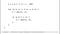Exponentiation by squaring on fixed point numbers

At DappHub, we’ve been thinking about the concept of safe unsigned integer arithmetic for a very long time and fixed-point mathematics in Solidity for even longer. Recently, we decided to package up a solution that should be useful to just about anyone working with Solidity smart contracts. Say hello to DS-Math! A component of our larger framework Dappsys, the `DSMath` type is meant to be a parent type of any contracts that you know will be performing arithmetic operations with unsigned integers. We really like the fact that its functions clearly describe what they are doing in an aesthetically-pleasing way (e.g. `add`, `sub`, `mul`, and `div` each safely add, subtract, multiply, and divide, respectively).

This may seem like a trivial feature, but in the world of smart contracts (and not coincidentally, NASA space shuttle programming) you can spend an entire day looking at ten lines of code. Within this context, clear and concise function definitions make it much easier to reason about the code’s logic and do a lot to preserve the programmer’s sanity. Additionally, the coverage of every arithmetic operation means you can instantly see when someone might be exposing themselves to a possible `uint` overflow simply by their use of raw arithmetic operators. If you ever see someone using raw arithmetic operators with unsigned integers in a smart contract, ask them why on earth they aren't using DS-Math like a decent, hardworking, responsible person.

# Introduction of Wad and Ray for advanced decimal math

An unfortunate characteristic of the modern day Solidity compiler is that it doesn’t support fixed-point mathematics yet. Thus, it is not currently possible to represent the number `3.1415` natively. Most projects workaround this feature by representing their decimal numbers as unsigned integers and specifying the level of precision that is being used alongside it. An example:

`// representing the number 121.234uint tokenBalance = 1212340000000000000000  uint decimals = 18`

However this means that it is no longer possible to multiply and divide these pseudo-decimal numbers using raw arithmetic operators! This is because of the disconnect between how integer arithmetic works and how decimal arithmetic is supposed to work. Consider this example:

`Regular decimal arithmetic:1.1 * 2.2 = 2.42Regular integer arithmetic adds orders of magnitude:110 * 220 = 24200`

This means that if `decimals` were set to `2`, as they would be in this case, the result will be `242.00` instead of the desired `2.42`. Clearly a solution is needed here.

Enter Wad and Ray mathematics. These are two new higher-level numerical types that are meant to correctly represent decimal numbers as unsigned integers by using arithmetic functions in `DSMath`. A Wad is a decimal number with 18 digits of precision and a Ray is a decimal number with 27 digits of precision. Both are stored in `uint128` variables.

We first created these numerical types out of necessity for the Maker project. Since Ether and most other ERC20 tokens use 18 digits of precision, we typically use Wads to represent token balances. Rays are more useful for extremely precise ratios that don’t go very high above zero. We use Rays in Maker for things like calculating accrued stability fees per second or making minute adjustments to Dai’s target price as the market price constantly fluctuates over time.

To see what makes these types useful, consider the above example using `wmul`, which returns the product of two Wads:

`Regular decimal arithmetic:1.1 * 2.2 = 2.42Regular integer arithmetic adds orders of magnitude:110 * 220 = 24200Wad arithmetic does not add orders of magnitude:wmul(1100000000000000000, 2200000000000000000) = 2420000000000000000`

Amazing! The Wad and Ray arithmetic functions of DS-Math make it a breeze to work with decimal numbers in Solidity.

# Using this package

If you would like to use DS-Math in your project, we recommend that you install our command line developer tool called dapp and simply run these commands:

`\$ dapp init\$ dapp install ds-math`

Otherwise you can just take the file and copy it anywhere. It is licensed under Apache 2.0, which is very permissive, so make whatever changes you like. Check out the official documentation for more details on using DS-Math and come say hello in our chat if you have any questions about this topic or any other aspect of programming smart contracts.

Written by

## DappHub

#### Free software for dapp devs

Welcome to a place where words matter. On Medium, smart voices and original ideas take center stage - with no ads in sight. Watch
Follow all the topics you care about, and we’ll deliver the best stories for you to your homepage and inbox. Explore
Get unlimited access to the best stories on Medium — and support writers while you’re at it. Just \$5/month. Upgrade